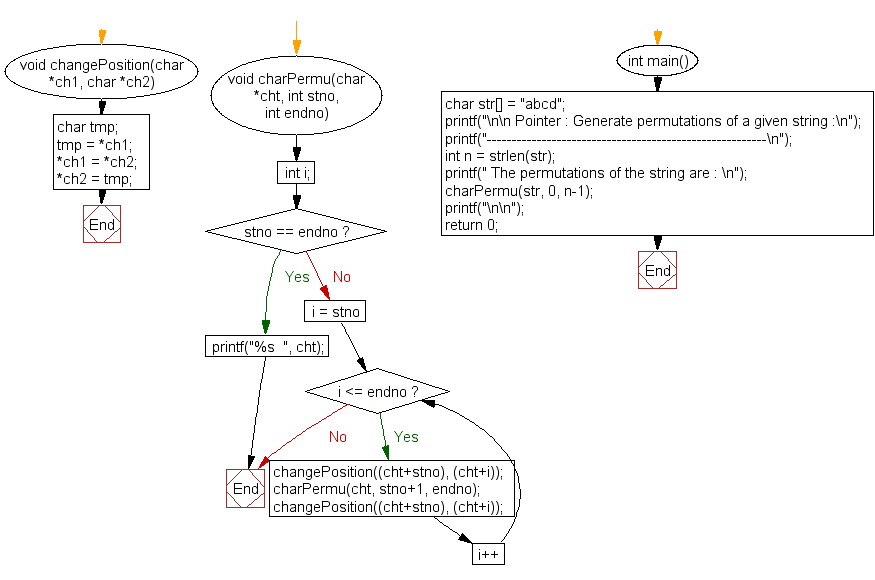﻿ C Program: Generate permutations of a given string - w3resource# C Exercises: Generate permutations of a given string

## C Pointer : Exercise-8 with Solution

Write a program in C to print all permutations of a given string using pointers.

Sample Solution:

C Code:

``````#include <stdio.h>
#include <string.h>

void changePosition(char *ch1, char *ch2)
{
char tmp;
tmp = *ch1;
*ch1 = *ch2;
*ch2 = tmp;
}
void charPermu(char *cht, int stno, int endno)
{
int i;
if (stno == endno)
printf("%s  ", cht);
else
{
for (i = stno; i <= endno; i++)
{
changePosition((cht+stno), (cht+i));
charPermu(cht, stno+1, endno);
changePosition((cht+stno), (cht+i));
}
}
}

int main()
{
char str[] = "abcd";
printf("\n\n Pointer : Generate permutations of a given string :\n");
printf("--------------------------------------------------------\n");
int n = strlen(str);
printf(" The permutations of the string are : \n");
charPermu(str, 0, n-1);
printf("\n\n");
return 0;
}
```
```

Sample Output:

``` Pointer : Generate permutations of a given string :
--------------------------------------------------------
The permutations of the string are :
ca  dbac  dcba  dcab  dacb  dabc
```

Flowchart:C Programming Code Editor:

Have another way to solve this solution? Contribute your code (and comments) through Disqus.

What is the difficulty level of this exercise?

Test your Programming skills with w3resource's quiz.

﻿

## C Programming: Tips of the Day

How to format strings using printf() to get equal length in the output?

You can specify a width on string fields, e.g.

`printf("%-20s", "initialization..."); `

And then whatever's printed with that field will be blank-padded to the width you indicate.

The - left-justifies your text in that field.

Ref : https://bit.ly/34DMOc3